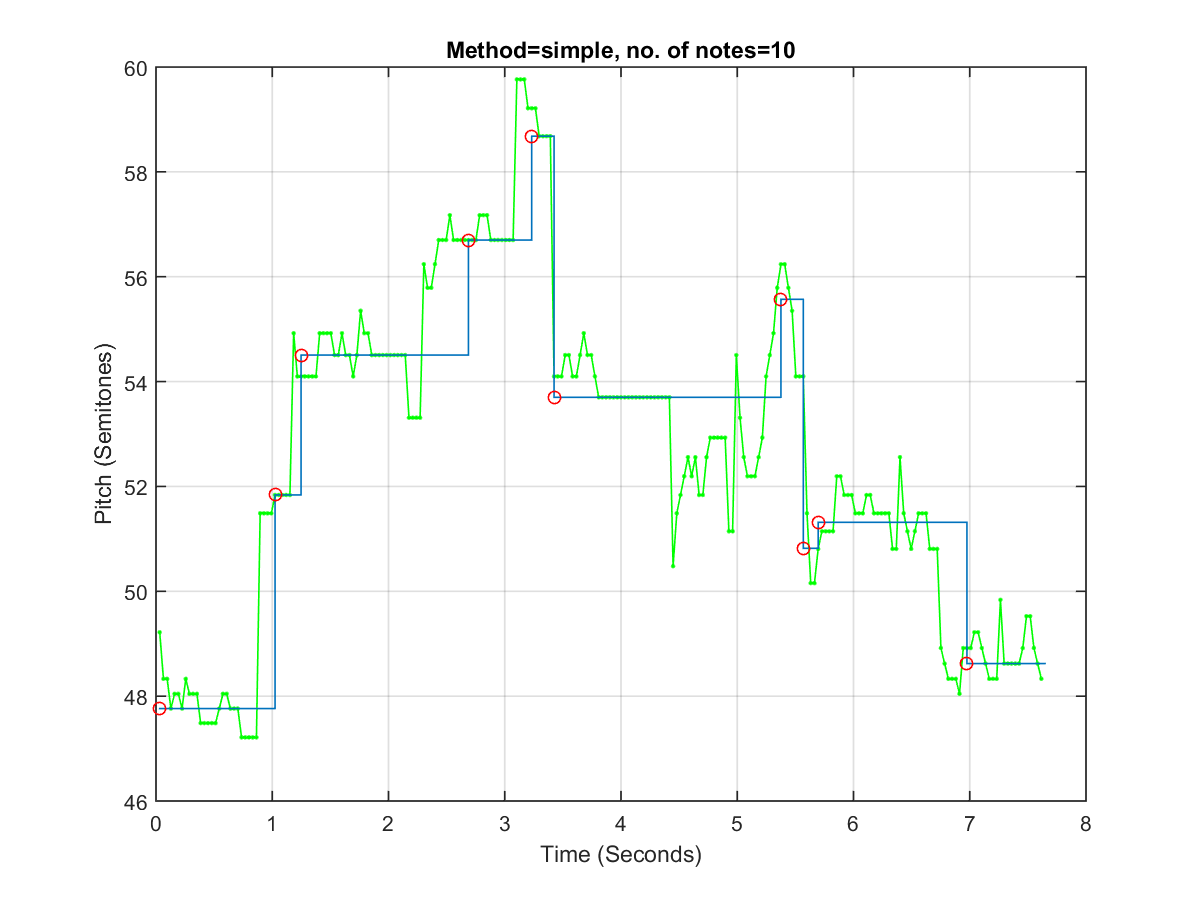## 14-7 LCS and Edit Distance

[chinese][all]

We can use note-based approach for melody recognition. First of all, we need to segment the input query pitch vector into music notes. Assume the input pitch vector is represented by pv(i), i=1~n, then the most straightforward method for note segmentation can be described as the pseudo code shown next:

for i=1:n
if |pv(i)-pv(i-1)|>q & the current note is long enough
Finish the current note and start a new note
else
Add pv(i) to the current note
end
end


The following example demonstrates the use of pv2note.m for note segmentation:

Example 1: noteSegment01.mpitchVector=[49.21841 48.33041 48.33041 47.76274 48.04425 48.04425 47.76274 48.33041 48.04425 48.04425 48.04425 47.48574 47.48574 47.48574 47.48574 47.48574 47.76274 48.04425 48.04425 47.76274 47.76274 47.76274 47.21309 47.21309 47.21309 47.21309 47.21309 51.48682 51.48682 51.48682 51.48682 51.83658 51.83658 51.83658 51.83658 51.83658 54.92247 54.09792 54.09792 54.09792 54.09792 54.09792 54.09792 54.92247 54.92247 54.92247 54.92247 54.50529 54.50529 54.92247 54.50529 54.50529 54.09792 54.50529 55.34996 54.92247 54.92247 54.50529 54.50529 54.50529 54.50529 54.50529 54.50529 54.50529 54.50529 54.50529 54.50529 53.31086 53.31086 53.31086 53.31086 56.23796 55.78827 55.78827 56.23796 56.69965 56.69965 56.69965 57.17399 56.69965 56.69965 56.69965 56.69965 56.69965 56.69965 56.69965 57.17399 57.17399 57.17399 56.69965 56.69965 56.69965 56.69965 56.69965 56.69965 56.69965 59.76274 59.76274 59.76274 59.21309 59.21309 59.21309 58.68037 58.68037 58.68037 58.68037 54.09792 54.09792 54.09792 54.50529 54.50529 54.09792 54.09792 54.50529 54.92247 54.50529 54.50529 54.09792 53.69992 53.69992 53.69992 53.69992 53.69992 53.69992 53.69992 53.69992 53.69992 53.69992 53.69992 53.69992 53.69992 53.69992 53.69992 53.69992 53.69992 53.69992 53.69992 53.69992 50.47805 51.48682 51.83658 52.19354 52.55803 52.19354 52.55803 51.83658 51.83658 52.55803 52.93035 52.93035 52.93035 52.93035 52.93035 51.14399 51.14399 54.50529 53.31086 52.55803 52.19354 52.19354 52.19354 52.55803 52.93035 54.09792 54.50529 54.92247 55.78827 56.23796 56.23796 55.78827 55.34996 54.09792 54.09792 54.09792 51.48682 50.15444 50.15444 50.80782 51.14399 51.14399 51.14399 51.14399 52.19354 52.19354 51.83658 51.83658 51.83658 51.48682 51.48682 51.48682 51.83658 51.83658 51.48682 51.48682 51.48682 51.48682 51.48682 50.80782 50.80782 52.55803 51.48682 51.14399 50.80782 51.14399 51.48682 51.48682 51.48682 50.80782 50.80782 50.80782 48.91732 48.62138 48.33041 48.33041 48.33041 48.04425 48.91732 48.91732 48.91732 49.21841 49.21841 48.91732 48.62138 48.33041 48.33041 48.33041 49.83678 48.62138 48.62138 48.62138 48.62138 48.62138 48.91732 49.52484 49.52484 48.91732 48.62138 48.33041]; opt=pv2note('defaultOpt'); opt.method='simple'; opt.minPitchError=0.8; opt.minNoteDuration=0.1; showPlot=1; note=pv2note(pitchVector, opt, showPlot); %fprintf('Hit return to hear the pitch vector...'); pause; fprintf('\n'); pvPlay(pitchVector, opt.frameRate); fs=8000; fprintf('Saving pitchVector.wav...\n'); wavwrite(pv2wave(pitchVector, opt.frameRate), fs, 8, 'pitchVector.wav'); %fprintf('Hit return to hear the segmented note...'); pause; fprintf('\n'); notePlay(note, 1); fprintf('Saving noteFromPv.wav...\n'); wavwrite(note2wave(note, 1, fs), fs, 8, 'noteFromPv.wav');Saving pitchVector.wav... [Warning: WAVWRITE will be removed in a future release. Use AUDIOWRITE instead.] [> In <a href="matlab:matlab.internal.language.introspective.errorDocCallback('wavwrite', 'E:\MATLAB\R2015a\toolbox\matlab\audiovideo\wavwrite.m', 48)" style="font-weight:bold">wavwrite</a> (<a href="matlab: opentoline('E:\MATLAB\R2015a\toolbox\matlab\audiovideo\wavwrite.m',48,0)">line 48</a>) In <a href="matlab:matlab.internal.language.introspective.errorDocCallback('noteSegment01', 'D:\users\jang\books\audioSignalProcessing\example\noteSegment01.m', 10)" style="font-weight:bold">noteSegment01</a> (<a href="matlab: opentoline('D:\users\jang\books\audioSignalProcessing\example\noteSegment01.m',10,0)">line 10</a>) In <a href="matlab:matlab.internal.language.introspective.errorDocCallback('goWriteOutputFile>dummyFunction', 'D:\users\jang\books\goWriteOutputFile.m', 85)" style="font-weight:bold">goWriteOutputFile>dummyFunction</a> (<a href="matlab: opentoline('D:\users\jang\books\goWriteOutputFile.m',85,0)">line 85</a>) In <a href="matlab:matlab.internal.language.introspective.errorDocCallback('goWriteOutputFile', 'D:\users\jang\books\goWriteOutputFile.m', 55)" style="font-weight:bold">goWriteOutputFile</a> (<a href="matlab: opentoline('D:\users\jang\books\goWriteOutputFile.m',55,0)">line 55</a>)] Saving noteFromPv.wav... [Warning: WAVWRITE will be removed in a future release. Use AUDIOWRITE instead.] [> In <a href="matlab:matlab.internal.language.introspective.errorDocCallback('wavwrite', 'E:\MATLAB\R2015a\toolbox\matlab\audiovideo\wavwrite.m', 48)" style="font-weight:bold">wavwrite</a> (<a href="matlab: opentoline('E:\MATLAB\R2015a\toolbox\matlab\audiovideo\wavwrite.m',48,0)">line 48</a>) In <a href="matlab:matlab.internal.language.introspective.errorDocCallback('noteSegment01', 'D:\users\jang\books\audioSignalProcessing\example\noteSegment01.m', 13)" style="font-weight:bold">noteSegment01</a> (<a href="matlab: opentoline('D:\users\jang\books\audioSignalProcessing\example\noteSegment01.m',13,0)">line 13</a>) In <a href="matlab:matlab.internal.language.introspective.errorDocCallback('goWriteOutputFile>dummyFunction', 'D:\users\jang\books\goWriteOutputFile.m', 85)" style="font-weight:bold">goWriteOutputFile>dummyFunction</a> (<a href="matlab: opentoline('D:\users\jang\books\goWriteOutputFile.m',85,0)">line 85</a>) In <a href="matlab:matlab.internal.language.introspective.errorDocCallback('goWriteOutputFile', 'D:\users\jang\books\goWriteOutputFile.m', 55)" style="font-weight:bold">goWriteOutputFile</a> (<a href="matlab: opentoline('D:\users\jang\books\goWriteOutputFile.m',55,0)">line 55</a>)]In fact, the method implemented by pv2note.m is the simplest way for note segmentation. Possible enhancements include:

1. Finish the current note whenever there is a rest.
2. If the singer has a good absolute pitch, we can round off each note to the nearest integer. On the other hand, if the singer has a good relative pitch, we can shift up and down to find a best shift amount that minimizes the absolute error.
3. We can also employ the concept of DP to find the best way for note segmentation, such that the difference between the original pitch vector and the segmented notes is minimized.

Once we finish note segmentation, we can use the note-based representation for melody recognition, as follows.

1. In the first method, we only consider the pitch difference between two neighboring notes, regardless of the note's absolute pitch and duration. For instance, if the note pitch is [69, 60, 58, 62, 62, 67, 69], we can convert it into a ternary string [D, D, U, S, U, U] where D (down), U (up) and S (same) are used to described the relationship between two neighboring notes. Once we have converted both the input query and the reference song into two ternary string vectors, we can invoke LCS (longest common subsequence) or ED (edit distance) to compute their distance.
2. In the second method, we use the note pitch directly, regardless of the note duration. For instance, if the note pitch is [69, 60, 58, 62, 62, 67, 69], we can simply invoke type-1 or type-2 DTW to compute the distance. Since we are using the note pitch directly, we need to perform key transposition before invoking DTW. On the other hand, if we are using the difference of the note pitch for comparison, then we do not need to invoke key transposition.
3. In the third method, we need to consider both note pitch and note duration. We can still use DTW-like method for comparison, except that we need to consider note pitch and note duration separately. For note pitch, we can take the difference to deal with key variation. For note duration, we can take the ratio of neighboring note duration to take care of tempo variation. We can then invoke DTW that compute the cost function as a weighted average of pitch and duration cost.

Audio Signal Processing and Recognition (音訊處理與辨識)